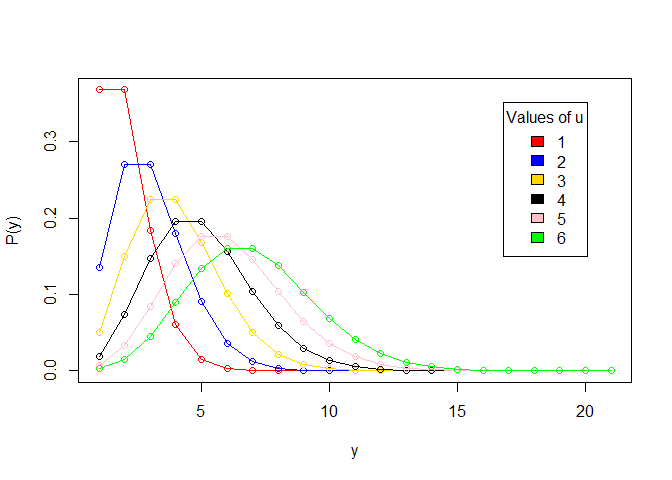# Define Poison regression?

It is used to predict the outcome variable which represents counts from the given set of continuous predictor variable.

Poisson Regression helps us analyze both count data and rate data by allowing us to determine which explanatory variables (X values) have an effect on a given response variable (Y value, the count or a rate). Poisson regression is used to model response variables (Y-values) that are counts. It tells you which explanatory variables have a statistically significant effect on the response variable. In other words, it tells you which X-values work on the Y-value.
Poisson regression is most commonly used to analyze rates, whereas logistic regression is used to analyze proportions. The chapter considers statistical models for counts of independently occurring random events, and counts at different levels of one or more categorical outcomes

The observations must be independent of one another. Mean=Variance By definition, the mean of a Poisson random variable must be equal to its variance. Linearity The log of the mean rate, log(λ ), must be a linear function of x.

Poisson distribution is a statistical theory named after French mathematician Siméon Denis Poisson. It models the probability of event or events y occurring within a specific timeframe, assuming that y occurrences are not affected by the timing of previous occurrences of y. This can be expressed mathematically using the following formula:

Here, μ (in some textbooks you may see λ instead of μ) is the average number of times an event may occur per unit of exposure. It is also called the parameter of Poisson distribution. The exposure may be time, space, population size, distance, or area, but it is often time, denoted with t. If exposure value is not given it is assumed to be equal to 1.

Let’s visualize this by creating a Poisson distribution plot for different values of μ.

First, we’ll create a vector of 6 colors:

``````# vector of colors
colors <- c("Red", "Blue", "Gold", "Black", "Pink", "Green")
``````

Next, we’ll create a list for the distribution that will have different values for μ:

``````# declare a list to hold distribution values
poisson.dist < - list()
</code>
``````

Then, we’ll create a vector of values for μ and loop over the values from μ each with quantile range 0-20, storing the results in a list:

``````a < - c(1, 2, 3, 4, 5, 6) # A vector for values of u
for (i in 1:6) {
poisson.dist[[i]] <- c(dpois(0:20, i)) # Store distribution vector for each corresponding value of u
}
</code>
``````

Finally, we’ll plot the points using `plot()`. `plot()` is a base graphics function in R. Another common way to plot data in R would be using the popular `ggplot2` package; this is covered in Dataquest’s R courses. But for this tutorial, we will stick to base R functions.

``````# plot each vector in the list using the colors vectors to represent each value for u
plot(unlist(poisson.dist), type = "o", xlab="y", ylab = "P(y)",
col = colors[i])
for (i in 1:6) {
lines(unlist(poisson.dist[i]), type = "o", col = colors[i])
}
# Adds legend to the graph plotted
legend("topright", legend = a, inset = 0.08, cex = 1.0, fill = colors, title = "Values of u")
``````Note that we used `dpois(sequence,lambda)` to plot the Probability Density Functions (PDF) in our Poisson distribution. In probability theory, a probability density function is a function that describes the relative likelihood that a continuous random variable (a variable whose possible values are continuous outcomes of a random event) will have a given value. (In statistics, a “random” variable is simply a variable whose outcome is result of a random event.)

A regression in practice fits coefficients to independent variables such that they construct a curve passing through the dependent variable. In a poisson regression, the dependent variable is a count variable. For example, the dependent variable might be the number of cars that cross a bridge every day across several months.

More abstractly, a poisson regression is a generalized linear model that uses a poisson distribution for the dependent variable. A generalized linear model is a model that can fit a range of distributions for the dependent variable — a normal distribution is used for linear regression, a binomial distribution is used for logistic regression, etc.